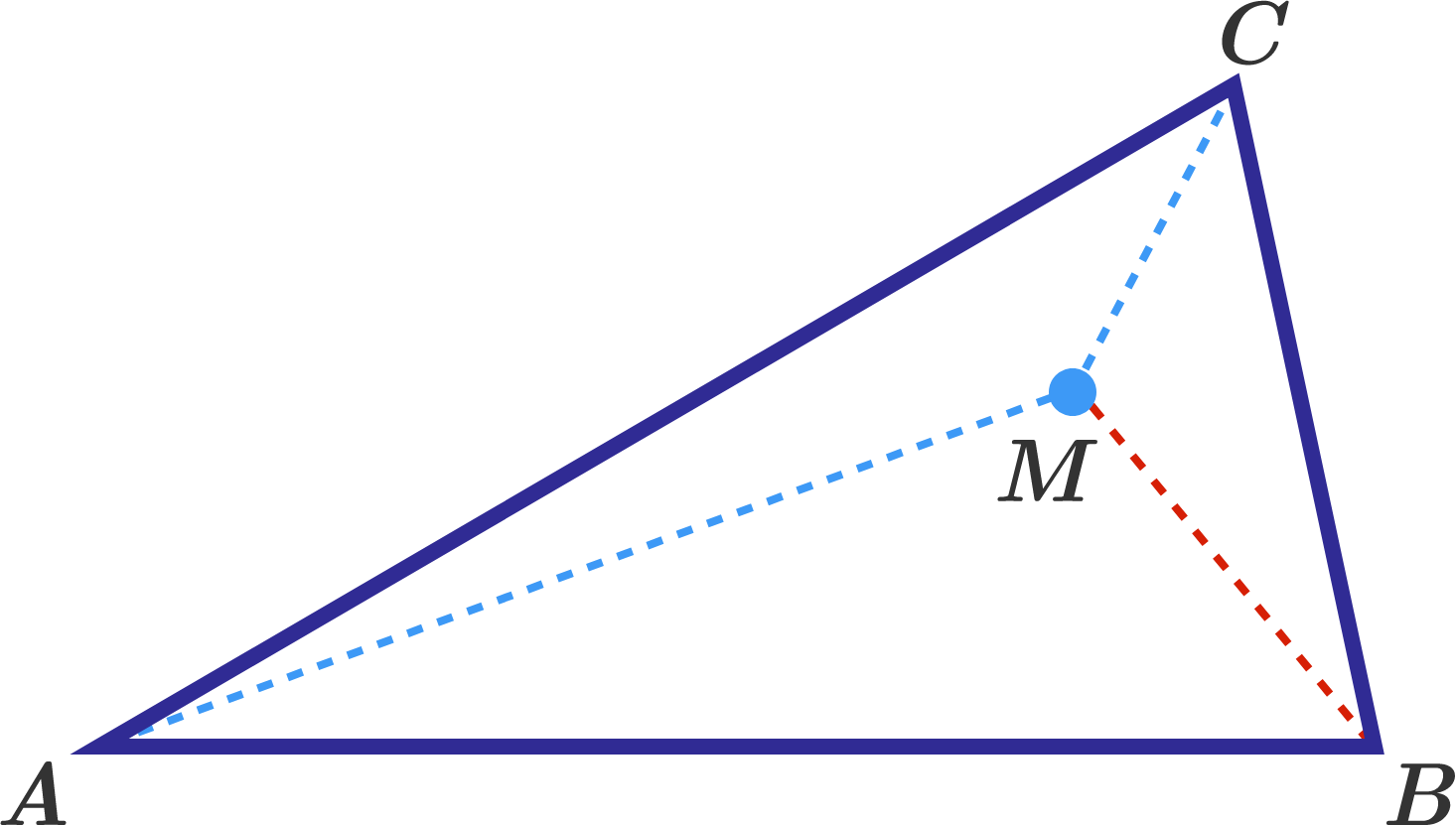# Too Many Angles, Too Little Information

Geometry Level 2In a triangle $ABC$, the measures of the angles are $\angle A=30^\circ,$ $\angle B=80^\circ.$ Point $M$ lies inside the triangle, such that $\angle MAC = 10^\circ,$ $\angle MCA = 30^\circ.$ What is the measure (in degrees) of $\angle BMC?$

×